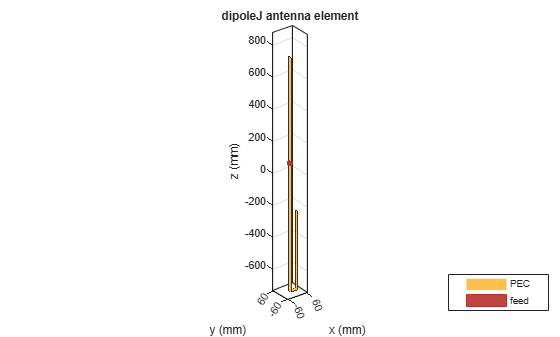# dipoleJ

Create J-dipole antenna

## Description

Use the `dipoleJ` object to create a J-dipole on the Y-Z plane. The antenna contains a half-wavelength radiator and a quarter-wavelength stub. By default, the antenna dimensions are for an operating frequency of 144 MHz.## Creation

### Syntax

``jdipole = dipoleJ``
``jdipole = dipoleJ(Name,Value)``

### Description

example

````jdipole = dipoleJ` creates a J-dipole antenna for an operating frequency of 144 MHz.```

example

````jdipole = dipoleJ(Name,Value)` creates a J-dipole antenna with additional properties specified by one or more name-value pair arguments. For example, `jdipole = dipoleJ('Width',0.2)` creates a J-dipole with a strip width of 0.2 m. Enclose each property name in quotes.```

## Properties

expand all

Radiator length, specified as a scalar in meters.

Example: `'RadiatorLength',0.9`

Example: `jdipole.RadiatorLength = 0.9`

Data Types: `double`

Parallel line stub length, specified as a scalar in meters.

Example: `'StubLength',0.3`

Example: `jdipole.StubLength = 0.3`

Data Types: `double`

Strip width, specified as a scalar in meters.

Example: `'StripWidth',0.0500`

Example: `jdipole.StripWidth = 0.0500`

Data Types: `double`

Space between the parallel line stub and the radiator, specified as a scalar in meters.

Example: `'Spacing',0.0500`

Example: `jdipole.Spacing = 0.0500`

Data Types: `double`

Signed distance to the feed from the base of stub on the large arm, specified as a scalar in meters.

Example: `'FeedOffset',0.0345`

Example: `jdipole.FeedOffset = 0.0345`

Data Types: `double`

Lumped elements added to the antenna feed, specified as a lumped element object handle. You can add a load anywhere on the surface of the antenna. By default, the load is at the origin. For more information, see `lumpedElement`.

Example: `'Load',lumpedelement`, where, `lumpedelement` is the object handle for the load created using `lumpedElement`.

Example: ```jdipole.Load = lumpedElement('Impedance',75)```

Tilt angle of the antenna, specified as a scalar or vector with each element unit in degrees. For more information, see Rotate Antennas and Arrays.

Example: `'Tilt',90`

Example: `ant.Tilt = 90`

Example: `'Tilt',[90 90]`,`'TiltAxis',[0 1 0;0 1 1]` tilts the antenna at 90 degrees about the two axes defined by the vectors.

Note

The `wireStack` antenna object only accepts the dot method to change its properties.

Data Types: `double`

Tilt axis of the antenna, specified as:

• Three-element vector of Cartesian coordinates in meters. In this case, each coordinate in the vector starts at the origin and lies along the specified points on the X-, Y-, and Z-axes.

• Two points in space, each specified as three-element vectors of Cartesian coordinates. In this case, the antenna rotates around the line joining the two points in space.

• A string input describing simple rotations around one of the principal axes, 'X', 'Y', or 'Z'.

Example: `'TiltAxis',[0 1 0]`

Example: `'TiltAxis',[0 0 0;0 1 0]`

Example: `ant.TiltAxis = 'Z'`

Note

The `wireStack` antenna object only accepts the dot method to change its properties.

Data Types: `double`

## Object Functions

 `show` Display antenna or array structure; display shape as filled patch `axialRatio` Axial ratio of antenna `beamwidth` Beamwidth of antenna `charge` Charge distribution on metal or dielectric antenna or array surface `current` Current distribution on metal or dielectric antenna or array surface `design` Design prototype antenna or arrays for resonance at specified frequency `EHfields` Electric and magnetic fields of antennas; Embedded electric and magnetic fields of antenna element in arrays `impedance` Input impedance of antenna; scan impedance of array `mesh` Mesh properties of metal or dielectric antenna or array structure `meshconfig` Change mesh mode of antenna structure `optimize` Optimize antenna or array using SADEA optimizer `pattern` Radiation pattern and phase of antenna or array; Embedded pattern of antenna element in array `patternAzimuth` Azimuth pattern of antenna or array `patternElevation` Elevation pattern of antenna or array `returnLoss` Return loss of antenna; scan return loss of array `sparameters` S-parameter object `vswr` Voltage standing wave ratio of antenna

## Examples

collapse all

Create and view a default J-dipole antenna.

`d = dipoleJ`
```d = dipoleJ with properties: RadiatorLength: 0.9970 StubLength: 0.4997 Spacing: 0.0460 Width: 0.0200 FeedOffset: -0.6994 Tilt: 0 TiltAxis: [1 0 0] Load: [1x1 lumpedElement] ```
`show(d)`Create and view a J-dipole antenna with the following specifications:

Stub length = 0.485 m

FeedOffset = 0.049 m

```dj = dipoleJ('RadiatorLength',0.978,'StubLength',0.485, ... 'FeedOffset',0.070); show(dj)```Calculate the impedance of the antenna over a frequency span 140MHz - 150MHz.

`impedance(dj,linspace(140e6,150e6,51));`